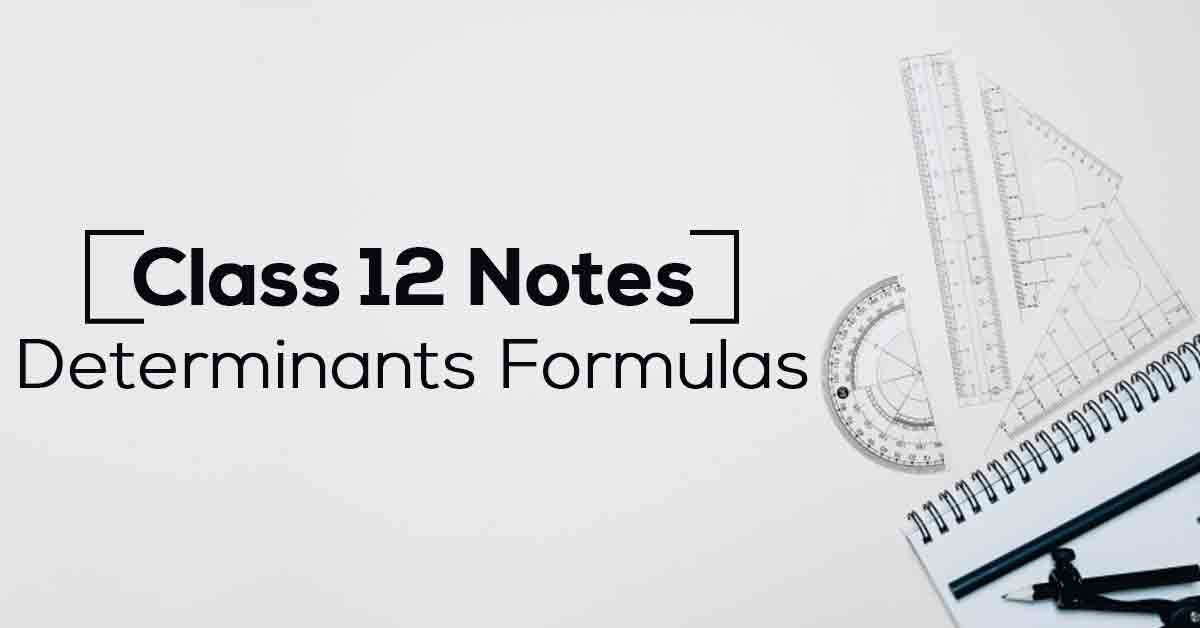Class 12th Math Determinants Formulas CBSE 2023

# Determinants Class 12 Notes# Chapter 4 Determinants Formulas

This article has all the important NCERT notes for Class 12 Math's Chapter 4. Students can follow these NCERT notes to prepare for their exams. The NCERT notes are an essential resource that helps students revise the entire chapter 4 in a short time. Designed as per the latest CBSE Syllabus, the NCERT notes can be extremely effective for exam preparation.

### Points to Remember

• If we interchange any two rows or two columns, the sign of determinants will change.

• If any two rows or two columns are identical, the value of determinants will be zero.

• A system of equations is consistent or inconsistent according to whether its solution exists or not.

• A square matrix A has an inverse if and only if A is singular.

• If we multiply each element of a row or a column of a determinant by constant k, then the value of the determinant is multiplied by k.

### Topics and Sub-topics

Since the students are new to Determinants, they may have some difficulty initially grasping the concept. However, once the students start practicing the Solutions for Class 12 Maths Chapter 4, things will become extremely easy. The NCERT notes can be the key to having a better understanding of the chapter.

Vidyakul provides notes and practice questions for all the subtopics of Class 12 Math Chapter 4. The learning module is designed as per the latest syllabus which can help students in their exam preparation. Students can practice the questions for free at Vidyakul to score good marks and prepare for the exams accordingly.

 Section Name Topic Name 4.1 Determinants 4.2 Properties of Determinants 4.3 Area of A Triangle 4.4 Minor and Cofactors 4.5 Adjoint and Inverse of A Matrix 4.6 Applications of Determinants and Matrices